Main Content

# recreg

Recursive linear regression

## Syntax

recreg(X,y)
recreg(Tbl)
recreg(___,Name,Value)
[Coeff,SE] = recreg(___)
recreg(ax,___)
[Coeff,SE,coeffPlots] = recreg(___)

## Description

recreg recursively estimates coefficients (β) and their standard errors in a multiple linear regression model of the form y = + ε by performing successive regressions using nested or rolling windows. recreg has options for OLS, HAC, and FGLS estimates, and for iterative plots of the estimates.

example

recreg(X,y) plots iterative coefficient estimates with ±2 standard error bands for each coefficient using the multiple linear regression model y = Xβ + ε.

example

recreg(Tbl) fits the data in the table Tbl to multiple linear regression model. The first numPreds columns are the predictors (X) and the last column is the response (y).

example

recreg(___,Name,Value) specifies options using one or more name-value pair arguments in addition to the input arguments in previous syntaxes. For example, you can specify the estimation method by using 'Estimator' or whether to include an intercept in the multiple regression model by using 'Intercept'.

example

[Coeff,SE] = recreg(___) returns the coefficient estimates and corresponding standard error estimates for each of the subsample regressions.
recreg(ax,___) plots on the axes specified in ax instead of the axes of new figures. The option ax can precede any of the input argument combinations in the previous syntaxes.
[Coeff,SE,coeffPlots] = recreg(___) additionally returns handles to plotted graphics objects. Use elements of coeffPlots to modify properties of the plots after you create it.

## Examples

collapse all

Apply recursive regressions using nested windows to look for instability in an explanatory model of real GNP for a period spanning World War II.

Load the Nelson-Plosser data set.

load Data_NelsonPlosser

The time series in the data set contain annual, macroeconomic measurements from 1860 to 1970. For more details, a list of variables, and descriptions, enter Description in the command line.

Several series have missing data. Focus the sample to measurements from 1915 to 1970. Identify the breakpoint index corresponding to 1945, the end of the war.

span = (1915 <= dates) & (dates <= 1970); bp = find(dates(span) == 1945);

Consider the multiple linear regression model

${\text{GNPR}}_{t}={\beta }_{0}+{\beta }_{1}{\text{IPI}}_{t}+{\beta }_{2}{\text{E}}_{t}+{\beta }_{3}{\text{WR}}_{t}.$

Collect the model variables into a tabular array. Position the predictors in the first three columns, and the response in the last column. Compute the number of coefficients in the model.

Mdl = DataTable(span,[4,5,10,1]); numCoeff = size(Mdl,2); % Three predictors and an intercept

Estimate the coefficients using recursive regressions, and return separate plots for the iterative estimates. Identify the iteration corresponding to the end of the war.

recreg(Mdl);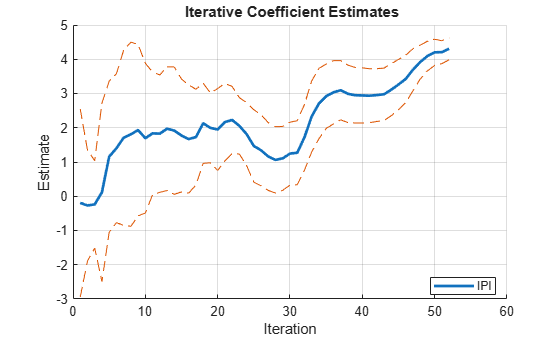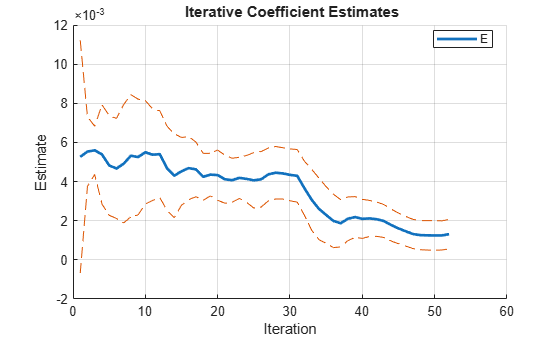bpIter = bp - numCoeff
bpIter = 27 

By default, recreg forms the subsamples using nested windows. The end of the war (1945) occurs at the 27th iteration.

All coefficients show some initial, transient instability during the "burn-in" period (see Tip). The plot of WR seems stable since the line is relatively flat. However, the plots of E, IPI, and the intercept (Const) show instability, particularly just after iteration 27.

Check coefficient estimates for instability in a model of food demand around World War II. Implement forward and backward recursive regressions in a rolling window.

Load the U.S. food consumption data set, which contains annual measurements from 1927 through 1962 with missing data due to the war.

load Data_Consumption

For more details on the data, enter Description at the command prompt.

Plot the series.

P = Data(:,1); % Food price index I = Data(:,2); % Disposable income index Q = Data(:,3); % Food consumption index figure; plot(dates,[P I Q],'o-') axis tight grid on xlabel('Year') ylabel('Index') title('{\bf Time Series Plot of All Series}') legend({'Price','Income','Consumption'},'Location','SE')Measurements are missing from 1942 through 1947, which correspond to World War II.

To examine elasticities, apply the log transformation to each series.

LP = log(P); LI = log(I); LQ = log(Q);

Consider a model in which log consumption is a linear function of the logs of food price and income. In other words,

${\text{LQ}}_{t}={\beta }_{0}+{\beta }_{1}{\text{LI}}_{t}+{\beta }_{2}\text{LP}+{\epsilon }_{t}.$

${\epsilon }_{t}$ is a Gaussian random variable with mean 0 and standard deviation ${\sigma }^{2}$.

Identify the breakpoint index at the end of the war, 1945. Ignore missing years with missing data.

numCoeff = 4; % Three predictors and an intercept T = numel(dates(~isnan(P))); % Sample size bpIdx = find(dates(~isnan(P)) >= 1945,1) - numCoeff
bpIdx = 12 

The 12th iteration corresponds to the end of the war.

Plot forward recursive-regression coefficient estimates using a rolling window of size 1/4 the sample size. Indicate to plot the coefficients of LP and LI only in the same figure.

X = [LP LI]; y = LQ; window = ceil(T*1/4); recreg(X,y,'Window',window,'Plot','combined','PlotVars',[0 1 1],... 'VarNames',{'Log-price' 'Log-income'});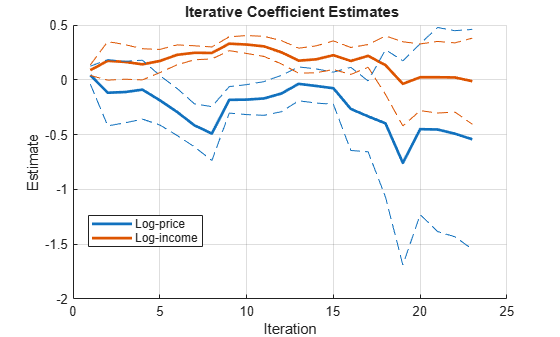Plot forward recursive-regression coefficient estimates using a rolling window of size 1/3 the sample size.

window = ceil(T*1/3); recreg(X,y,'Window',window,'Plot','combined','PlotVars',[0 1 1],... 'VarNames',{'Log-price' 'Log-income'});Plot forward recursive-regression coefficient estimates using a rolling window of size 1/2 the sample size.

window = ceil(T*1/2); recreg(X,y,'Window',window,'Plot','combined','PlotVars',[0 1 1],... 'VarNames',{'Log-price' 'Log-income'});As the window size increases, the lines show less volatility, but the coefficients do exhibit instability.

If a linear regression model violates classical linear model assumptions, then OLS coefficient standard errors are incorrect. However, recreg has options to estimate coefficients and standard errors that are robust to heteroscedastic or autocorrelated innovations.

Simulate a series from this piecewise regression model with AR(1) errors whose regression coefficient changes at time 51.

$\left\{\begin{array}{c}\begin{array}{l}{y}_{t}=5+3{x}_{t}+{u}_{t}\\ {u}_{t}=0.6{u}_{t-1}+{\epsilon }_{t}\end{array};t=1,...,50\\ \begin{array}{l}{y}_{t}=5-{x}_{t}+{u}_{t}\\ {u}_{t}=0.6{u}_{t-1}+{\epsilon }_{t}\end{array};t=51,...,100\end{array}.$

${\epsilon }_{t}$ is a series of Gaussian innovations with mean 0 and standard deviation 0.5. ${x}_{t}$ is Gaussian with mean 1 and standard deviation 0.25.

rng(1); % For reproducibility T = 100; muX = 1; sigmaX = 0.25; x = sigmaX*randn(T,1) + muX; ar = 0.6; sigma = 0.5; c = 5; b = [3 -1]; y = zeros(T,1); Mdl1 = regARIMA('AR',ar,'Variance',sigma,'Intercept',c,'Beta',b(1)); y(1:T/2) = simulate(Mdl1,T/2,'X',x(1:T/2)); Mdl2 = regARIMA('AR',ar,'Variance',sigma,'Intercept',c,'Beta',b(2)); y((T/2 + 1):T) = simulate(Mdl2,T/2,'X',x((T/2 + 1):T));

Estimate recursive regression coefficients using OLS.

[CoeffOLS,SEOLS] = recreg(x,y,'Plot','separate');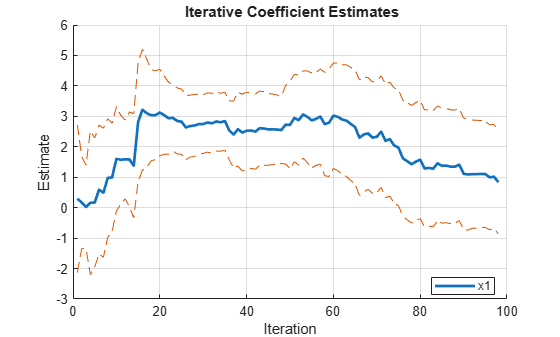After transient effects, 5 is within the confidence bounds of the intercept estimates. There is an insignificant, but persistent shock at iteration 50. The coefficient estimates show the structural change after iteration 60.

To account for autocorrelated innovations, estimate recursive regression coefficients using OLS, but with Newey-West robust standard errors. For estimating the HAC standard errors, use the quadratic-spectral weighting scheme.

hacOptions.Weights = 'QS'; [CoeffNW,SENW] = recreg(x,y,'Estimator','hac','Options',hacOptions,... 'Plot','separate');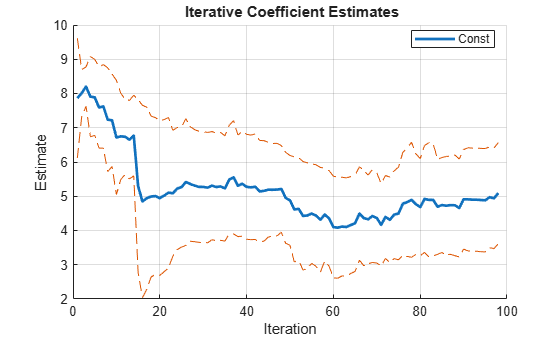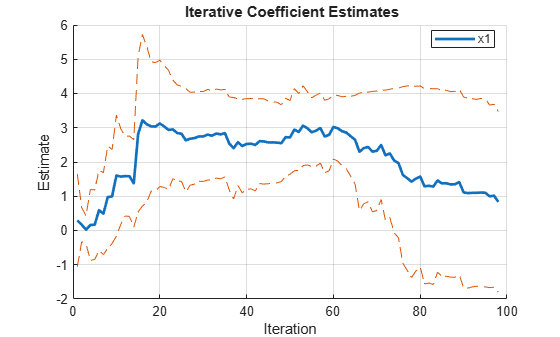The HAC coefficient estimates are the same as the OLS estimates. The confidence bounds are slightly different because the standard error estimators are different.

## Input Arguments

collapse all

Predictor data for the multiple linear regression model, specified as a numObs-by-numPreds numeric matrix.

numObs is the number of observations and numPreds is the number of predictor variables.

Data Types: double

Response data for the multiple linear regression model, specified as a numObs-by-1 numeric vector.

Data Types: double

Combined predictor and response data for the multiple linear regression model, specified as a numObs-by-numPreds + 1 tabular array.

The first numPreds columns of Tbl are the predictor data, and the last column is the response data.

Data Types: table

Axes on which to plot, specified as a vector of Axes objects with length equal to the number of plots specified by the Plot and PlotVars name-value pair arguments.

By default, recreg creates a separate figure for each plot.

Note

recreg removes observations with missing (NaN) values in the predictors or the response.

### Name-Value Pair Arguments

Specify optional comma-separated pairs of Name,Value arguments. Name is the argument name and Value is the corresponding value. Name must appear inside quotes. You can specify several name and value pair arguments in any order as Name1,Value1,...,NameN,ValueN.

Example: 'Intercept',false,'Estimator','fgls' indicates to exclude an intercept term from the regression model and to use FGLS to estimate coefficients and standard errors.

Indicate whether to include an intercept when recreg fits the regression model, specified as the comma-separated pair consisting of 'Intercept' and true or false.

ValueDescription
truerecreg includes an intercept when fitting the regression model. numCoeffs = numPreds + 1.
falserecreg does not include an intercept when fitting the regression model. numCoeffs = numPreds.

Example: 'Intercept',false

Data Types: logical

Window length, specified as the comma-separated pair consisting of 'Window' and a numeric scalar.

• To compute estimates using nested windows, do not specify 'Window'. In this case, recreg begins with the first numCoeffs + 1 observations, and then adds one observation at each iteration. The number of iterations is numIter = numObsnumCoeffs.

• To compute estimates using a rolling window, specify a window length. In this case, recreg shifts by one observation at each iteration. Window must be at least numCoeffs + 1 and no greater than numObs. The number of iterations is numIter = numObsWindow + 1.

Example: 'Window',10

Data Types: double

Estimation method, specified as the comma-separated pair consisting of 'Estimator' and a value in this table.

ValueDescription
'ols'

Ordinary least squares

'hac'

Heteroscedasticity and autocorrelation consistent (HAC) standard errors

'fgls'

Feasible generalized least squares coefficients and standard errors

Values 'hac' and 'fgls' call hac and fgls, respectively, with Name,Value argument settings specified by Options.

Example: 'Estimator','fgls'

Data Types: char | string

hac and fgls Name,Value argument names and corresponding values, specified as the comma-separated pair consisting of 'Options' and a structure array.

Use 'Options' to set any Name,Value argument except 'VarNames', 'Intercept', 'Display', or 'Plot'. For these options, see corresponding recreg Name,Value arguments.

By default, recreg calls hac or fgls using defaults. If Estimator is 'ols', then recreg ignores Options.

Example: 'Options',struct('ARLags',2) includes two lags in the AR innovations model for FGLS estimators.

Data Types: struct

Iteration direction, specified as the comma-separated pair consisting of 'Direction' and 'forward' or 'backward'.

ValueDescription
'forward'

Forward recursions move the window of observations from the beginning of the data to the end.

'backward'

Backward recursions first reverse the order of observations, and then implement forward recursions.

Example: 'Direction','backward'

Data Types: char | string

Flag indicating whether to plot test results, specified as the comma-separated pair consisting of 'Plot' and 'separate', 'combined', or 'off'. Plots show iterative coefficient estimates with ±2 standard error bands.

ValueDescription
'separate'Produces separate figures for each coefficient
'combined'Combines all plots in a single set of axes
'off'Turns off all plotting

The defaults are:

• 'off' when recreg returns output arguments

• 'separate' otherwise

Example: 'Plot','off'

Data Types: char | string

Flags indicating which coefficients to plot, specified as the comma-separated pair consisting of 'PlotVars' and a logical vector of length numCoeffs. The first element corresponds to Intercept, if present, followed by indicators for each of the numPred predictors in X or Tbl. The default is true(numCoeffs,1) to plot all coefficients.

Example: 'PlotVars',[false true true false]

Data Types: logical

Variable names for plotted coefficients, specified as the comma-separated pair consisting of 'VarNames' and a string vector or cell vector of names. The length is either numPreds or the number of selected predictors in PlotVars (that is, sum(PlotVars)). If Intercept is true, then recreg adds the name 'Const' to VarNames. Defaults are {'x1','x2',...} for the matrix X and Tbl.Properties.VariableNames for the tabular array Tbl.

Data Types: cell | string

## Output Arguments

collapse all

Coefficient estimates for each subsample regression, returned as a numCoeffs-by-numIter numeric matrix. The first row contains the intercept, if present, followed by rows for predictor coefficients in the column order of X or Tbl. Window determines numIter, the number of columns.

Standard error estimates for each subsample regression, returned as a numCoeffs-by-numIter numeric matrix. Row order and number of columns correspond to Coeff.

Handles to plotted graphics objects, returned as a vector of graphics objects. coeffPlots contains unique plot identifiers, which you can use to query or modify properties of the plot.

coeffPlots is not available if the value of the Plot name-value pair argument is 'off'.

## Tips

Plots of nested-window estimates typically show volatility during a “burn-in” period, in which the number of subsample observations is only slightly larger than the number of coefficients in the model. After this period, any further volatility is evidence of coefficient instability. Sudden changes in coefficient values can indicate a structural change, and sustained changes can indicate model misspecification. For structural change tests, see cusumtest and chowtest.

 Enders, W. Applied Econometric Time Series. New York: John Wiley & Sons, Inc., 2009.

 Johnston, J. and J. DiNardo. Econometric Methods. New York: McGraw Hill, 1997.

Download ebook